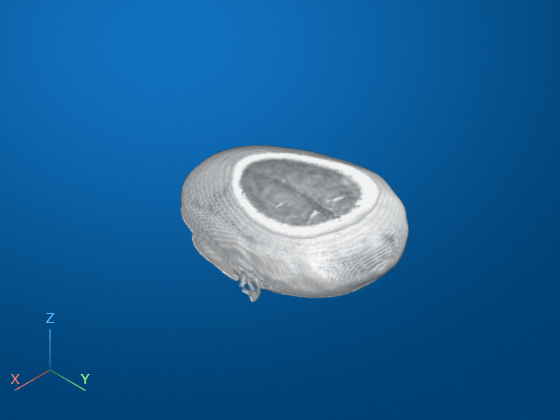# imrotate3

Rotate 3-D volumetric grayscale image

## Syntax

``B = imrotate3(V,angle,W)``
``B = imrotate3(V,angle,W,method)``
``B = imrotate3(V,angle,W,method,bbox)``
``B = imrotate3(___,"FillValues",fillValues)``

## Description

example

````B = imrotate3(V,angle,W)` rotates the 3-D volume `V` by `angle` degrees counterclockwise around an axis passing through the origin ```[0 0 0]```. `W` is a 1-by-3 vector which specifies the direction of the axis of rotation in 3-D space. By default, `imrotate3` sets the values of voxels in `B` that are outside the boundaries of the rotated volume to `0`.```
````B = imrotate3(V,angle,W,method)` also specifies the interpolation method.```
````B = imrotate3(V,angle,W,method,bbox)` also specifies the size of the output volume, `bbox`. If you specify `"crop"`, then `imrotate3` makes the output volume the same size as the input volume. If you specify `"loose"`, then `imrotate3` makes the output volume large enough to include the entirety of the rotated volume.```
````B = imrotate3(___,"FillValues",fillValues)` sets the fill values used for output pixels without a corresponding pixel in the input image.```

## Examples

collapse all

Load and display 3-D volumetric grayscale image.

```load("mri"); vol = squeeze(D); volshow(vol);```Rotate the volume 90 degrees around the Z axis.

`volRot = imrotate3(vol,90,[0 0 1],"nearest","loose");`

Display the rotated output volume. You can also explore the volume in the Volume Viewer app.

`volshow(volRot);`## Input Arguments

collapse all

Volume to be rotated, specified as a 3-D numeric array, 3-D logical array, or 3-D categorical array.

Data Types: `single` | `double` | `int8` | `int16` | `int32` | `int64` | `uint8` | `uint16` | `uint32` | `uint64` | `logical` | `categorical`

Rotation angle in degrees, specified as numeric scalar. To rotate the volume clockwise, specify a negative value for `angle`. `imrotate3` makes the output volume `B` large enough to contain the entire rotated 3-D volume.

Data Types: `single` | `double` | `int8` | `int16` | `int32` | `int64` | `uint8` | `uint16` | `uint32` | `uint64`

Direction of the axis of rotation in 3-D space in Cartesian coordinates, specified as a 1-by-3 numeric vector.

If you want to specify the direction of the axis of rotation in spherical coordinates, use `sph2cart` to convert values to Cartesian coordinates before passing it to `imrotate3`.

Example: `[0 0 1]` rotates the volume around the Z axis

Data Types: `single` | `double` | `int8` | `int16` | `int32` | `int64` | `uint8` | `uint16` | `uint32` | `uint64` | `logical`

Interpolation method, specified as one of the following values.

MethodDescription
`"nearest"`

Nearest neighbor interpolation. The output voxel is assigned the value of the voxel that the point falls within. No other voxels are considered.

Nearest-neighbor interpolation is the only method supported for categorical images and it is the default method for images of this type.

`"linear"`

Trilinear interpolation.

Trilinear interpolation is the default method for numeric and logical images.

`"cubic"`

Tricubic interpolation

Note

Tricubic interpolation can produce pixel values outside the original range.

Data Types: `char` | `string`

Size of the output volume, specified as either of the following values.

MethodDescription
`"crop"`

Make the output volume the same size as the input volume, cropping the rotated volume to fit.

`"loose"`

Make the output volume large enough to contain the entire rotated volume. Usually, the rotated volume is larger than the input volume.

Data Types: `char` | `string`

Fill values used for output pixels outside the input image, specified as one of the following values. `imrotate3` uses fill values for output pixels when the corresponding inverse transformed location in the input image is completely outside the input image boundaries.

Image Type

Format of Fill Values

Numeric image or logical image
• Numeric scalar. The default fill value of numeric and logical images is `0`.

Categorical image
• Valid category in the image, specified as a string scalar or character vector.

• `missing`, which corresponds to the `<undefined>` category. `missing` is the default fill value for categorical images. For more information, see `missing`.

Data Types: `single` | `double` | `int8` | `int16` | `int32` | `int64` | `uint8` | `uint16` | `uint32` | `uint64` | `logical` | `char` | `string`

## Output Arguments

collapse all

Rotated volume, returned as a numeric, logical, or categorical array of the same class as the input volume, `V`.

## Tips

• `imrotate3` assumes that the input volume `V` is centered on the origin `[0 0 0]`. If your volume is not centered on the origin, then use `imtranslate` to translate the volume to `[0 0 0]` before using `imrotate3`. You can translate the output volume `B` back to the original position with the opposite translation vector.

## Version History

Introduced in R2017a

expand all